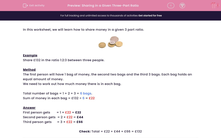# Sharing in a Given Three-Part Ratio

In this worksheet, students share sums of money in the given three-part ratios.This content is premium and exclusive to EdPlace subscribers.Key stage:  KS 2

Curriculum topic:   Maths and Numerical Reasoning

Curriculum subtopic:   Ratio and Proportion

Difficulty level:#### Worksheet Overview

In this worksheet, we will learn how to share money in a given 3 part ratio.Example

Share £132 in the ratio 1:2:3 between three people.

Method

The first person will have 1 bag of money, the second two bags and the third 3 bags. Each bag holds an equal amount of money.

We need to work out how much money there is in each bag.

Total number of bags = 1 + 2 + 3 = 6 bags.

Sum of money in each bag = £132 ÷ 6 = £22

First person gets       = 1 × £22 = £22

Second person gets  = 2 × £22 = £44

Third person gets      = 3 × £22 = £66

Check: Total = £22 + £44 + £66 = £132

### What is EdPlace?

We're your National Curriculum aligned online education content provider helping each child succeed in English, maths and science from year 1 to GCSE. With an EdPlace account you’ll be able to track and measure progress, helping each child achieve their best. We build confidence and attainment by personalising each child’s learning at a level that suits them.

Get started